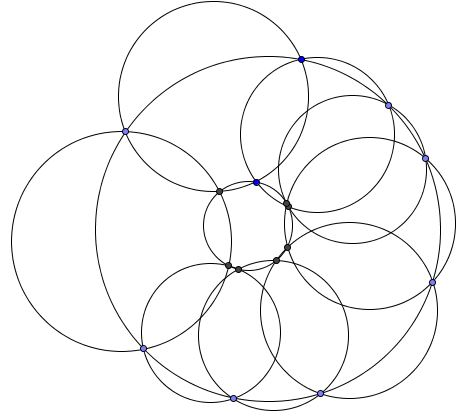# Two Quadruplets of Concyclic Points

### Problem

Four circles $S_1,$ $S_2,$ $S_3,$ $S_4$ cross pairwise: $S_1\cap S_2=\{A_1,B_1\},$ $S_2\cap S_3=\{A_2,B_2\},$ $S_3\cap S_4=\{A_3,B_3\},$ $S_4\cap S_1=\{A_4,B_4\}.$ Assume points $A_i,\space n=1,2,3,4$ are concyclic.Then points points $A_i,\space n=1,2,3,4$ are also concyclic. (The circles involved may have infinite radius, i.e. be straight lines.)

### Solution

The problem admits an equivalent formulation:

Given six points, $A_1,$ $A_3,$ $B_4,$ $B_3,$ $B_1,$ $A_2,$ assume that the circumcircles $(A_1A_2A_3),$ $(A_3B_3B_4),$ $(A_1B_1B_4)$ concur at point $A_4.$Prove that the circumcircles $(A_1A_2B_1),$ $(A_2A_3B_3),$ $(B_1B_3B_4)$ concur at point $B_2.$

But this is Miquel's theorem for circles.

### Acknowledgment

This is problem 217b from I. M. Yaglom's Geometric Transformations, v II, Russian original (1956). I'd guess that it is also included in a relatively late (2009) translation Geometric Transformations IV, but I do not have the book.

A more elementary solution has been placed on a separate page.

### A Second Look

The problem could be approached differently (I change notation):

Given two quadruplets of concyclic points $\{A_i\}$, $\{B_i\},$ $i=1,2,3,4'$ It is known that points $A_1,B_1,B_2,A_2$ are concyclic and so are points $A_2,B_2,B_3,A_3$ and $A_3,B_3,B_4,A_4.$ Prove that points $A_4,B_4,B_1,A_1$ are also concyclic.

In this formulation the problem admits a generalization:

Given two sets of $n$ concyclic points each $\{A_i\}$, $\{B_i\},$ $i=1,\ldots,n,$ where n is an even integer. It is known that points $A_i,B_i,B_{i+1},A_{i+1}$ are concyclic for $i=1,\ldots ,n-1.$ Prove that points $A_n,B_n,B_1,A_1$ are also concyclic.

This is especially obvious when the circumcircles of points $\{A_i\}$ and $\{B_i\}$ are concyclic. In this case, the quadrilaterals $A_iB_iB_{i+1}A_{i+1}$ are isosceles trapezoids, and, for even $n,$ so is $A_nB_nB_1A_1.$Since every pair of non-intersecting circles could be inverted into concyclic ones, the statement is validated for any two non-intersecting circles.

### Inversion - Introduction